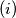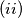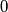### IMO Shortlist 2018 problem C6

Kvaliteta:
Avg: 0,0
Težina:
Avg: 8,0

Let$a$ and$b$ be distinct positive integers. The following infinite process takes place on an initially empty board.$(i)$ If there is at least a pair of equal numbers on the board, we choose such a pair and increase one of its components by$a$ and the other by$b$.$(ii)$ If no such pair exists, we write two times the number$0$.

Prove that, no matter how we make the choices in$(i)$, operation$(ii)$ will be performed only finitely many times.

Izvor: https://www.imo-official.org/problems/IMO2018SL.pdf